# Microeconomics - Demand | Supply and Demand | Microeconomics Help

## Economics Basics: Demand & Supply

The market is an amazing instrument, it enables people who have never met and who know nothing about each other to interact and do business. Supply & demand is perhaps one of the most fundamental concepts of economics and it is the backbone of a market economy.

• Demand refers to how much (quantity) of a product or service is desired by buyers. The quantity demanded is the amount of a product people are willing to buy at a certain price; the relationship between price and quantity demanded is known as the demand relationship.
• Supply represents how much the market can offer. The quantity supplied refers to the amount of a certain good producers are willing to supply when receiving a certain price. The correlation between price and how much of a good or service is supplied to the market is known as the supply relationship.

### Concept of demand:

• One of the most important building blocks of economic analysis is the concept of demand. Demand is the quantity of a good or service needed or desired by the consumer to consume and the ability and willingness to pay for it at a given point of time.
• Demand is recorded in a demand schedule and is plotted on a graph as an inverse downward sloping demand curve. The demand schedule tells the quantity of goods / services that a consumer is willing and would be able to buy at specific prices at a given point. This relationship between quantities demanded and price is called the demand relationship.

### The Law of Demand:

(1). The law of demand states that when the price of a good rises, the amount demanded falls, and when the price falls, the amount demanded rises.

(2). The law of demand states that, if all other factors remain equal, the higher the price of a good, the smaller is the quantity demanded; and the lower the price of a good, the greater is the quantity demanded.

(3). This law holds true because as the price of a goods increases the real income with the consumer declines and in order to avoid forgoing consumption of other important goods the consumer decides to consume lesser quantity of the good.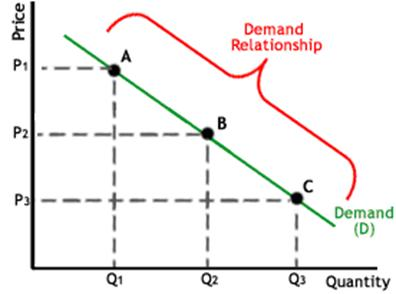The above figure shows the demand relationship with the help of the demand curve. We can see that at point A when the price is highest at P1 quantity demanded is lowest at Q1. As price decreases from P1 to P2 and P3, the quantity demanded increases to Q2 and Q3 respectively as shown at the point B and C. The graph illustrates the demand relationship which explains that as P increases the demand at points (A, B and C) in the market decreases hence the Q decreases. This demonstrates a down-ward slope.

### The Demand Curve:

We know that, the quantity demanded of a good usually is a strong function of its price. Demand is illustrated by the demand curve and the demand schedule. The term quantity demanded referes to a point on a demand curve.

By definition, the demand curve displays quantity demanded as the independent variable (the X axis) and price as the dependent variable (the Y axis). For basic analysis, the demand curve often is approximated as a straight line. A movement refers to a change along a curve. On the demand curve, a movement denotes a change in both price and quantity demanded from one point to another on the curve.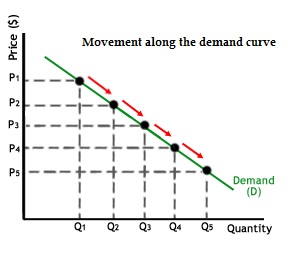### Determinants of demand:

Various factors affect the quantity demanded by a consumer of a good or service. A number of factors may influence the demand for a products. The key determinants of demand are as follows:-

• Price of the good: This is the most important determinant of demand. The relationship between price of the good and quantity demanded is generally inverse as we will see later while studying law of demand.

• Price of related goods:

• Substitutes: If the price of a substitute goes down than the quantity demanded of the good also goes down and vice versa.

• Complements : If the price of gasoline goes up the quantity demanded of automobiles will go down. An increase in the price of a complement reduces demand. Thus the price of complements have a inverse relationship with the demand of a good.

• Income: Higher the income of the consumer the more will be quantity demanded of the good. The only exception to this will be inferior goods whose demand decreases with an increase in income level.

• Individual taste and preferences: A preference for a particular good may affect the consumer’s choice and he / she may continue to demand the same even in rising prices scenario.

• Expectations about future prices & income: If the consumer expects prices to rise in future he / she may continue to demand higher quantities even in a rising price scenario and vice versa.

### Assumptions of the law:

Like every law, the law of demand also has its own limitation. We have mentioned, while stating the law of demand above, the assumptions that other things remaining same or unchanged.

In real life this is not possible but for theoretical purposes and for analysing the relationship between demand and price we take the assumption of all other things to be unchanged. Few of the key assumptions which we assume to be unchanged while stating the law of demand are:

1. Prices of other goods (substitutes & complements) remain unchanged.
2. Tastes and preferences remain constant.
3. Income level of the consumer does not increase or decrease.
4. The commodity is a normal good and has no status value.
5. Consumer expectations are neutral or they do not expect rise / fall in the price of the good.

### Exceptions to the law of demand:

Unlike other laws, law of demand also have few exceptions i.e. there is no inverse relationship between price and quantity demanded for these goods. Few of them are as follows:

• Giffen Goods : These are those inferior goods whose quantity demanded decreases with decrease in price of the good. This can be explained using the concept of income effect and substitution effect as discussed earlier. In case of such goods the positive income effect is higher than the negative substitution effect resulting is an overall positive (direct) relationship between price and quantity demanded.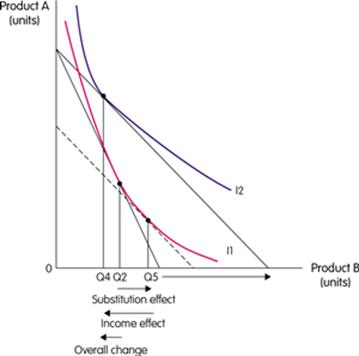As shown in the above figure, the substitution effect due to the decrease in price increase the quantity demanded from Q2 to Q5, while the income effect gives more disposable income which leads to decrease in quantity demanded from Q5 to Q4. So the net effect is a decrease in quantity demanded from Q2 to Q4.

• Commodities which have snob value or regarded as status symbols: Expensive commodities like jewellery, AC cars, etc., are used to define status and to display one’s wealth. These goods doesn't follow the law of demand and quantity demanded increases with price rise as more expensive these goods become, more will be their worth as a status symbol.
• Expectation of change in the price of the goods in future: If a consumer expects the price of a good to increase in future, it may start accumulating greater amount of the goods for future consumption even at the presently increased price. The same holds true vice versa.

## Difference between movement or shifts along the demand curve

Changes in demand for a commodity can be shown through the demand curve in two ways namely:

• (1)- Movement along the demand curve.
• (2)- Shifts of the demand curve.

The change in the demand of a commodity due to change in its price leads to moving the demand curve upward or downward depending upon the change in price. When the price rises, the demand falls. And when the price falls the demand for that commodity rises leading to movement in the demand curve.

Shift in the demand curve is the result of the price remaining constant but the demand changing due to several other factors such as, change in fashion, income, and population, etc.

### Movement along the demand curve:

A movement along a demand curve is defined as a change in the quantity demanded due to changes in the price of a good will result in a movement along the demand curve. For instance, a fall in the price of apples from P1 to P2 causes an increase in the quantity demanded from Q1 to Q2.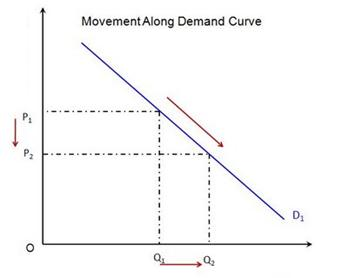In other words, a movement occurs when a change in the quantity demanded is caused only by a change in price, and vice versa.

### Shifts in the demand curve:

A shift of the demand curve is referred to as a change in demand due any factor other than price. A demand curve will shift if any of these occurs:

1. Change in the price of other goods (complements and substitutes); leading to increase / decrease of real income.
2. Change in the income level.
3. Change in consumers’ taste and preferences.
4. Change in expectations.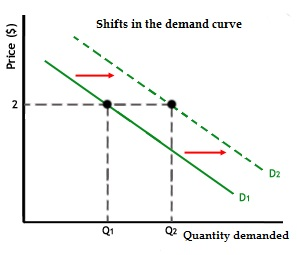Each of these factors tends the demand curve to shift downwards to the left or upwards to the right. While downward shift signifies decrease in demand, an upward shift of the demand curve shows an increase in the demand. For example, if there is a positive news report about FMCG products, the quantity demanded at each price may increase, as demonstrated by the demand curve shifting to the right. There are many factors may influence the demand for a product, and changes in one or more of those factors may cause a shift in the demand curve.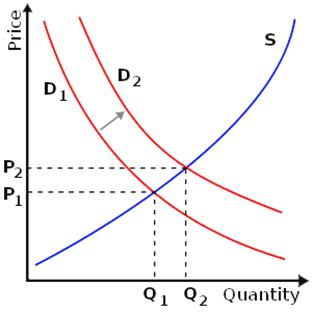An outward (rightward) shift in demand increases both equilibrium price and quantity.

As shown in the demand curve if any of these changes factors changes, the demand curve will shift to D2 from D1 and accordingly the price and quantity demanded will change. Movements along a demand curve is the result of increase or decrease of the price of the good, while the demand curve shifts when any demand determinant other than price changes.

To Submit Microeconomics - Demand, Demand curve, and related assignments/problems Click here

Microeconomics | Microeconomics Help | Economics Basics: Demand & Supply | Concept Of Demand | Demand Curve | Microeconomics Theories | Aggregate Demand | Law of Demand | Movement Along The Demand Curve | Shifts In The Demand Curve | Principle Of Microeconomics | Demand Definition | Define Demand | Demand Analysis | Demand Function | Demand Forecasting | supply and demand | Online tutoring